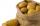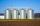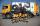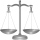Potatoes

For three days the store sold 1400 kg of potatoes. The first day they sold 100 kilograms of potatoes less than the second day, the third-day three-fifths of what they sold the first day. How many kgs of potatoes sold every day?

Result

a =  500
b =  600
c =  300

Solution:

a+b+c=1400
a = b-100
c = 3/5*a

a+b+c = 1400
a-b = -100
3a-5c = 0

a = 500
b = 600
c = 300

Calculated by our linear equations calculator.

Leave us a comment of this math problem and its solution (i.e. if it is still somewhat unclear...):Be the first to comment!To solve this verbal math problem are needed these knowledge from mathematics:

Do you have a system of equations and looking for calculator system of linear equations?

Next similar math problems:

1. Potatoes bagsI have three bags with 21 kg potatoes. The first bag is 5.5 kg more than in the second bag and the third is 0.5 kg more than in the second bag. Determine how many kgs of potatoes is in each bag.
2. WarehousesIn the three warehouses, a total of 70 tons of grain was stored. In the second warehouse was stored 8.5t less and in the third 3.5t more than in the first. How many tons of grain was stored in each warehouse?
3. Three workshopsThere are 2743 people working in three workshops. In the second workshop works 140 people more than in the first and in third works 4.2 times more than the second one. How many people work in each workshop?
4. Three friendsDanica, Lenka and Dalibor have altogether 96 kg. Lenka weighs 75% more than Dalibor and Danica weighs 6 kg more than Dalibor. Determine the weight of Danice, Lenka and Dalibor.
5. Three unknownsSolve the system of linear equations with three unknowns: A + B + C = 14 B - A - C = 4 2A - B + C = 0
6. Theorem proveWe want to prove the sentence: If the natural number n is divisible by six, then n is divisible by three. From what assumption we started?
7. Collecting dayAt collecting day at school pupils of three classes took total of 1063 kg of paper. Class B collected half more than A Class and class C 55 kg more than B. How many kg of paper collected each class?
8. BottlesThe must is sold in 5-liter and 2-liter bottles. Mr Kucera bought a total of 216 liters in 60 bottles. How many liters did Mr. Kucera buy in five-liter bottles?
9. FamilyFamily has 4 children. Ondra is 3 years older than Matthew and Karlos 5 years older than the youngest Jane. We know that they are together 30 years and 3 years ago they were together 19 years. Determine how old the children are.
10. Vegan shopTo the shops brought together 23.2 kg of goods, fruits, vegetables and nuts. fruit was 4.7 kg more than vegetables, nuts was 1.5 kg less than the fruit. Determine the amount of fruits, vegetables and nuts.
11. SavingsPaul has a by half greater savings than half Stanley, but the same savings as Radek. Staney save 120 CZK less than Radek. What savings have 3 boys together?
12. Trio weightAdelka, Barunka, and Cecilka are weight in pairs. Adelka with Barunka weighs 98 kg, Barunka with Cecilka 90 kg and Adelka with Cecilka 92 kg. How much does each of them weigh?
13. LegsCancer has 5 pairs of legs. The insect has 6 legs. 60 animals have a total of 500 legs. How much more are cancers than insects?
14. Elimination methodSolve system of linear equations by elimination method: 5/2x + 3/5y= 4/15 1/2x + 2/5y= 2/15
15. Linsys2Solve two equations with two unknowns: 400x+120y=147.2 350x+200y=144
16. Alcohol mixingHow much 55% alcohol we must pour into 1500 g 80% alcohol to form a 60% alcohol? How much 60% alcohol created?
17. ChildrenThe group has 42 children. There are 4 more boys than girls. How many boys and girls are in the group?